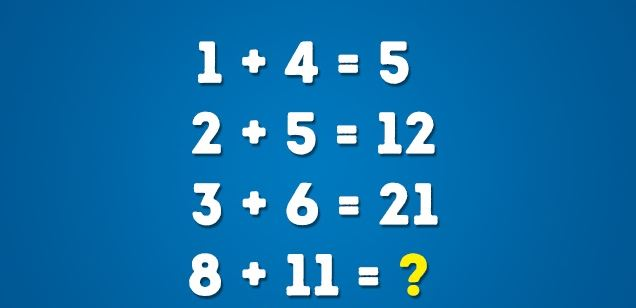# Almost Everyone is Failing This Simple Math Problem, Can You Solve it?

1028

This math problem has baffled Facebook and almost everyone is getting a simple answer.

It has gone viral with millions of likes and comments, yet there’s really no agreement. Try it and see what you’ll get.This problem has two possible solutions.

Solution #1:

Add the solution of the previous equation to the numbers on the next line:

1 + 4 = 5
5 + 2 + 5 = 12
12 + 3 + 6 = 21

Here’s what we get: 21 + 8 + 11 = 40.

Solution #2:

Now try to multiply the numbers as shown below:

1 + (4 × 1) = 5
2 + (5 × 2) = 12
3 + (6 × 3) = 21

Here’s what we get: 8 + (8 × 11) = 96.